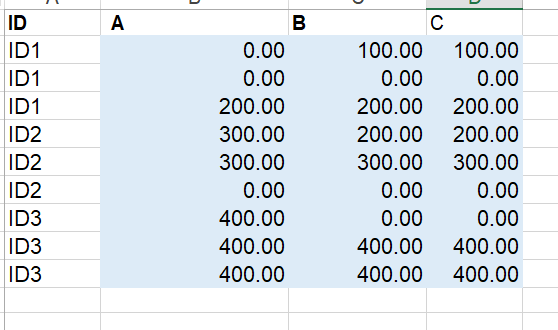# Excel value checking with different data tables sharing the same unique ID

Hi All,

I have been stuck in this excel checking for so long and your help or any idea input.

1. Table 1 and table 2 shares the same unique value and can be cross-checed. However, I need to sum amount1 based on value, after that, I need to compared the sum value with table 2 - amount 2. Apart from sum value, value also needs to be matched with each unique ID.

2. Table 3 and 4 is more challenging to me.
Firstly the value of sum of table 3 need to be compared against the value of sum in table 4 for each unique ID. However, the value for table C is under column name but the value for table D is from the row variables.

Please let me know if additional information required, thank you so much.DataTable reference.xlsx (11.2 KB)

Hi,

Please get the data of all the sheets using read Range so that you have an individual datatable for each sheet. Now you can join the datatables as you prefer like datatable from sheet1 with datatable from sheet2 with their columnNames (ID) so you get a consolidated datatable as a result which has both Amount1 and Amount2 and you can do the calculations as required by iterating through the consoldated table.

Hope this would help

Thanks, I think table 1 and 2 can use this method.

For table 3 and 4, it is quite different and I need to add value based on column and row variables. Is there any additional function I can use?